# Cube in a sphere

The cube is inscribed in a sphere with volume 7253 cm3. Determine the length of the edges of a cube.

Correct result:

a =  13.9 cm

#### Solution:

$V=7253 \ \text{cm}^3 \ \\ V=\dfrac{ 4 }{ 3 } \pi r^3 \ \\ \ \\ r=\sqrt{ \dfrac{ 3 \cdot \ V }{ 4 \pi } }=\sqrt{ \dfrac{ 3 \cdot \ 7253 }{ 4 \cdot \ 3.1416 } } \doteq 12.0082 \ \text{cm} \ \\ \ \\ D=2 \cdot \ r=2 \cdot \ 12.0082 \doteq 24.0163 \ \text{cm} \ \\ \ \\ u=D=24.0163 \doteq 24.0163 \ \text{cm} \ \\ \ \\ u=\sqrt{ 3 } a \ \\ \ \\ a=u/\sqrt{ 3 }=24.0163/\sqrt{ 3 }=13.9 \ \text{cm}$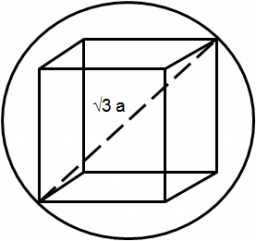Our examples were largely sent or created by pupils and students themselves. Therefore, we would be pleased if you could send us any errors you found, spelling mistakes, or rephasing the example. Thank you!

Please write to us with your comment on the math problem or ask something. Thank you for helping each other - students, teachers, parents, and problem authors.Math student
i am good at this

2 years ago  2 LikesCrazy Butterfly
This is easy.Tips to related online calculators
Need help calculate sum, simplify or multiply fractions? Try our fraction calculator.
Do you want to convert length units?
Tip: Our volume units converter will help you with the conversion of volume units.
Pythagorean theorem is the base for the right triangle calculator.

#### You need to know the following knowledge to solve this word math problem:

We encourage you to watch this tutorial video on this math problem:

## Next similar math problems:

• EightEight small Christmas balls with a radius of 1 cm have the same volume as one large Christmas ball. What has a bigger surface: eight small balls, or one big ball?
• Wall thicknessThe hollow metal ball has an outside diameter of 40 cm. Determine the wall thickness if the weight is 25 kg and the metal density is 8.45 g/cm3.
• KostkaKostka je vepsána do koule o poloměru r = 6 cm. Kolik procent tvoří objem kostky z objemu koule?
• Volume ratio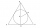Calculate the volume ratio of balls circumscribed (diameter r) and inscribed (diameter ϱ) into an equilateral rotating cone.
• Gas sphere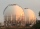The gas tank has the shape of a sphere with a diameter of 14 m. How many m3 of gas will fit in it?
• Clay balls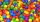How many clay balls with a radius of 1 cm can be made from a ball of clay with a radius of 8 cm?
• The water tankThe water tank has the shape of a sphere with a radius of 2 m. How many liters of water will fit in the tank? How many kilograms of paint do we need to paint the tank, if we paint with 1 kg of paint 10 m2?
• What is bigger?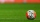Which ball has a larger volume: a football with a circumference of 66 cm or a volleyball with a diameter of 20 cm?
• CannonballsOf the three cannonballs with a diameter of 16 cm, which landed in the castle courtyard during the battle, the castle blacksmith cast balls with a diameter of 10 cm, which fit into the cannons placed on the walls. How many cannonballs did the blacksmith c
• What percentageWhat percentage of the Earth’s surface is seen by an astronaut from a height of h = 350 km. Take the Earth as a sphere with the radius R = 6370 km
• Two hemispheres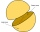In a wooden hemisphere with a radius r = 1, a hemispherical depression with a radius r/2 was created so that the bases of both hemispheres lie in the same plane. What is the surface of the created body (including the surface of the depression)?
• The EarthThe Earth's surface is 510,000,000 km2. Calculates the radius, equator length, and volume of the Earth, assuming the Earth has the shape of a sphere.
• Spherical segmentCalculate the volume of a spherical segment 18 cm high. The diameter of the lower base is 80 cm, the upper base 60 cm.
• Planet Earth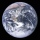What is the weight of the planet Earth, if its average density is ρ = 2.5 g/cm ^ 3?
• Hemisphere cutCalculate the volume of the spherical layer that remains from the hemisphere after the 3 cm section is cut. The height of the hemisphere is 10 cm.How many times does the surface of a sphere decrease if we reduce its radius twice?The spherical cap has a base radius of 8 cm and a height of 5 cm. Calculate the radius of a sphere of which this spherical cap is cut.